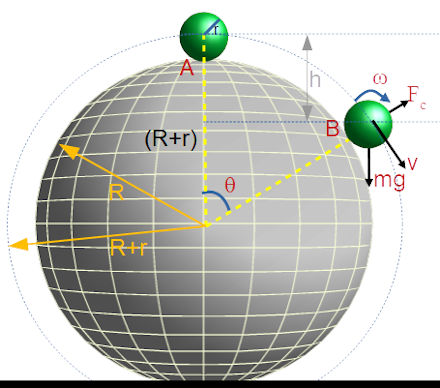# A uniform sphere of radius r starts rolling down without slipping from the top of another fixed...

## Question:

A uniform sphere of radius r starts rolling down without slipping from the top of another fixed sphere of radius R. Find the angular velocity of the sphere of radius r at the instant when it leaves contact with the surface of the fixed sphere.

## Rotational Kinetic Energy and Gravitational Potential Energy:

Rotational Kinetic Energy

Rotational kinetic energy of a rotating body is given by;

{eq}K.E. = \dfrac{1}{2} I \omega^2 {/eq}

where,

• {eq}I {/eq} is the moment of inertia of the body about the axis of rotation.
• {eq}\omega {/eq} is the angular velocity of the body about the axis of rotation.

Gravitational Potential Energy

Gravitational Potential Energy of an object near an Earth's surface is given by;

{eq}P.E. = mgh {/eq}

where,

• m is the mass of the object.
• g is the acceleration due to gravity.
• h is the height of the object from the reference point.

Let

• Mass of the small sphere is m.
• The angular speed of the small sphere when it leaves contact with the surface of the fixed sphere is {eq}\omega {/eq}
• The linear speed of the small sphere when it leaves contact with the surface of the fixed sphere is {eq}v {/eq}.
• {eq}\theta {/eq} is the angle made by the position vector of the small sphere with the vertical when it leaves contact with the surface of the fixed sphere.

Given:

• The radius of the small sphere is r.
• The radius of the fixed sphere is R.

The free-body diagram of the small sphere when it leaves contact with the surface of the fixed sphere is shown in the figure below.In the above figure,

• {eq}mg {/eq} is the weight of the small sphere.

{eq}F_c {/eq} is the centrifugal force on the small sphere.

If the small sphere leaves the contact with the surface of the fixed sphere at point B then;

{eq}\begin{align} F_c &= mg\cos\theta\\ \implies \dfrac{mv^2}{(R+r)} &= mg\cos\theta\\ \implies \dfrac{v^2}{(R+r)} &= g\cos\theta\ \ \ \ \ \ \ \ \ \ \ \ \ \ \ \ \ \ \ \ \ \ \ \ \ \ \ \ \ \ \ \text{Eqn. 1}\\ \end{align} {/eq}

As the small sphere rolling without slipping so, {eq}v = \omega R {/eq}.

The momentum of inertia of the small sphere about its own axis is {eq}I = \dfrac{2}{5}mr^2 {/eq}. (Treating the small sphere as a solid sphere.)

Potential energy of the small sphere at point B is {eq}P.E._{B} = 0 {/eq}

Kinetic energy of the small sphere at point A is {eq}K.E._{A}=0 {/eq}

Potential energy of the small sphere at point A is {eq}P.E._{A} =mgh= mg(R+r)(1-\cos\theta) {/eq}

Kinetic energy of the small sphere at point B is {eq}K.E._{B}=\dfrac{1}{2}mv ^2+\dfrac{1}{2}I\omega ^2 {/eq}

From the conservation of mechanical energy, the mechanical energy of the small sphere at point A is equal to the mechanical energy at point B.

{eq}\begin{align} K.E._{A} +P.E._{A} &= K.E._{B} +P.E._{B}\\ \implies 0 + mg(R+r)(1-\cos\theta) &= (\dfrac{1}{2}mv ^2+\dfrac{1}{2}I\omega ^2)+0 \\ \implies mg(R+r)(1-\cos\theta) &= (\dfrac{1}{2}mv ^2+\dfrac{1}{2}\times (\dfrac{2}{5}mr^2)\times (\dfrac{v }{r})^2)\\ \implies g(R+r)(1-\cos\theta) &= \dfrac{7}{10}v ^2\ \ \ \ \ \ \ \ \ \ \ \ \ \ \ \ \ \ \ \ \ \ \ \ \ \ \ \ \ \ \ \text{Eqn. 2}\\ \end{align} {/eq}

From equation 1 and 2,

{eq}v = \sqrt{\dfrac{10(R+r)g}{17}} {/eq}

Therefore, the angular velocity of the small sphere at the instant when it leaves contact with the surface of the fixed sphere is;

{eq}\omega = \dfrac{v}{r}=\sqrt{\dfrac{10(R+r)g}{17r^2}} {/eq}